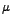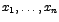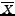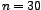DATA MINING
Desktop Survival Guide
by Graham Williams## Textual Summaries

The summary function provides the first insight into how the values for each variable are distributed:

 > summary(wine) Type Alcohol Malic Ash Alcalinity 1:59 Min. :11.03 Min. :0.740 Min. :1.360 Min. :10.60 2:71 1st Qu.:12.36 1st Qu.:1.603 1st Qu.:2.210 1st Qu.:17.20 3:48 Median :13.05 Median :1.865 Median :2.360 Median :19.50 Mean :13.00 Mean :2.336 Mean :2.367 Mean :19.49 3rd Qu.:13.68 3rd Qu.:3.083 3rd Qu.:2.558 3rd Qu.:21.50 Max. :14.83 Max. :5.800 Max. :3.230 Max. :30.00 Magnesium Phenols Flavanoids Nonflavanoids Min. : 70.00 Min. :0.980 Min. :0.340 Min. :0.1300 1st Qu.: 88.00 1st Qu.:1.742 1st Qu.:1.205 1st Qu.:0.2700 Median : 98.00 Median :2.355 Median :2.135 Median :0.3400 Mean : 99.74 Mean :2.295 Mean :2.029 Mean :0.3619 3rd Qu.:107.00 3rd Qu.:2.800 3rd Qu.:2.875 3rd Qu.:0.4375 Max. :162.00 Max. :3.880 Max. :5.080 Max. :0.6600 Proanthocyanins Color Hue Dilution Min. :0.410 Min. : 1.280 Min. :0.4800 Min. :1.270 1st Qu.:1.250 1st Qu.: 3.220 1st Qu.:0.7825 1st Qu.:1.938 Median :1.555 Median : 4.690 Median :0.9650 Median :2.780 Mean :1.591 Mean : 5.058 Mean :0.9574 Mean :2.612 3rd Qu.:1.950 3rd Qu.: 6.200 3rd Qu.:1.1200 3rd Qu.:3.170 Max. :3.580 Max. :13.000 Max. :1.7100 Max. :4.000 Proline Min. : 278.0 1st Qu.: 500.5 Median : 673.5 Mean : 746.9 3rd Qu.: 985.0 Max. :1680.0 

Next, we would like to know how the data is distributed. For categorical variables this will be how many of each level there are. For numeric variables this will be the mean and median, the minimum and maximum values, and an idea of the spread of the values of the variable.

We would also like to know about missing values (referred to in R as NAs--short for Not Available), and the summary function will also report this:

 > load("survey.RData") > summary(survey) [...] Native.Country Salary.Group United-States:29170 <=50K:24720 Mexico : 643 >50K : 7841 Philippines : 198 Germany : 137 Canada : 121 (Other) : 1709 NA's : 583 

We also see here that the categorical variable Native.Country has more than five levels, and there are 1,709 entities with values for this variable other than the five listed here. The five listed are the most frequently occurring.

The http://en.wikipedia.org/wiki/meanmean provides a measure of the average or central tendency of the data. It is denoted asifis the whole population (population mean), andif it is a sample of the population (sample mean).

In calculating the http://en.wikipedia.org/wiki/meanmean of a sample from a population we generally need at least 30 observations in the sample before it makes sense. This is based on the central limit theorem that indicates that forthe shape of a distribution approaches normal.

R provides the mean function to calculate the mean. The mean is also reported as part of the output from summary. The summary function in fact will use the method associated with the data type of the object passed. For example, if it is a data frame the function summary.data.frame will be called upon. To see the actual function definition, simply type the function name at the command line (without brackets). The actual code will be printed out. A user can then fine tune the function, if desired.

A quick trick to roughly get the mode of a dataset is to use the denisity.

 mode <- function (n) { n <- as.numeric(n) n.density <- density(n) round(n.density$x[which(n.density$y==max(n.density\$y))]) } 

You can then simply write your own functions to summarise the data using sapply:

 > sapply(wine, function(x) { x <- as.numeric(x) res <- c(mean(x), median(x), mode(x), mad(x), sd(x)) names(res) <- c("mean", "median", "mode", "mad", "sd") res }) Type Alcohol Malic Ash Alcalinity Magnesium Phenols mean 1.938202 13.0006180 2.336348 2.366517 19.494944 99.74157 2.295112 median 2.000000 13.0500000 1.865000 2.360000 19.500000 98.00000 2.355000 mode 2.000000 14.0000000 2.000000 2.000000 19.000000 90.00000 3.000000 mad 1.482600 1.0081680 0.770952 0.237216 3.039330 14.82600 0.748713 sd 0.775035 0.8118265 1.117146 0.274344 3.339564 14.28248 0.625851 Flavanoids Nonflavanoids Proanthocyanins Color Hue Dilution mean 2.0292697 0.3618539 1.5908989 5.058090 0.9574494 2.6116854 median 2.1350000 0.3400000 1.5550000 4.690000 0.9650000 2.7800000 mode 3.0000000 0.0000000 1.0000000 3.000000 1.0000000 3.0000000 mad 1.2379710 0.1260210 0.5633880 2.238726 0.2446290 0.7709520 sd 0.9988587 0.1244533 0.5723589 2.318286 0.2285716 0.7099904 Proline mean 746.8933 median 673.5000 mode 553.0000 mad 300.2265 sd 314.9075 

In the following sections we provide graphic presentations of the mean and standard variation.